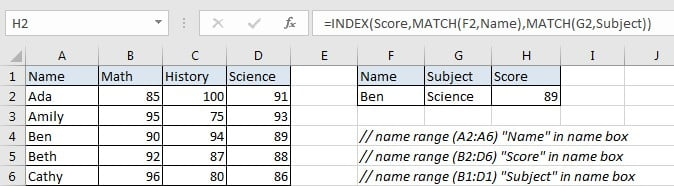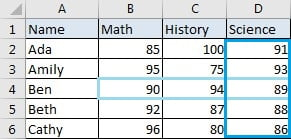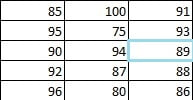# Basic Usage of INDEX & MATCH – Exact Match

In Excel, INDEX function and MATCH function are often used together for returning value or cell reference or range reference from specified position. And MATCH function is one of Excel lookup & reference functions that can perform approximate match or exact match by setting different match type values.

Today, we will show you how to use INDEX and MATCH to perform an exact match. This formula can also help you to retrieve score from a given table based on two conditions. If you meet similar scenarios in your daily work, you can directly use this formula to deal with your problem.

## EXAMPLEIn this example, we want to define a formula in H2 that can return score correctly based on the two entries of name and subject. This formula can retrieve data at the intersection of “Ben” and “Science” from range B2:D6. In this example, as the returned score must be included in range B2:D6, so this formula can perform an exact match and return score of science for Ben properly.

Before creating a formula to solve this problem, to illustrate the formula clearly, we defined three named ranges “Name” (A2:A6), “Score” (B2:D6) and “Subject” (B1:D1). In this article, to approach our goal, we will apply INDECT & MATCH functions together to perform an exact match.

## ANALYSIS

To return proper score based on the two entries of name and subject:

1. There are two conditions to determine the returned score: entered name (Ben) and subject (Science).

2. In this example, both the two entries “Ben” and “Science” can be found in the given table (A1:D6), so we can find their row and column numbers in this table, and with the help of row and column numbers, we can find out the intersection of them.

3. This formula can find out and return the intersection of “Ben” and “Science”.

4. This formula can retrieve data at the intersection.

To meet above conditions, we can apply INDEX and MATCH functions together. In Excel, INDEX and MATCH functions are good partners for retrieving data from a specified position. MATCH function can return the relative position from a range based on searching for the input values, and INDEXT can query the corresponding data according to the position. In this example, MATCH function returns the intersection of scores of “Ben” and scores of “Science”, then INDEX function returns the score at the intersection.

Above all, we create below formula to solve this problem:

`=INDEX(Score,MATCH(F2,Name),MATCH(G2,Subject))`

## FORMULA

Input formula =INDEX(Score,MATCH(F2,Name),MATCH(G2,Subject)) into H2, press ENTER, verify that “89” is returned properly.The intersection is D4, the data in D4 is 89.## FUNCTION INTRODUCTION

a. INDEX function returns a value or a reference based on given cell or range reference.

For array form:

Syntax:

`=INDEX(array, row_num, [column_num])`

For reference form:

Syntax:

`=INDEX(reference, row_num, [column_num], [area_num])`

There are two forms of INDEX functions. When applying INDEX with reference form, INDEX returns reference of the intersection of a particular row and column. You can apply array form if the first argument to INDEX is an array constant.

b. MATCH function returns the relative position of a cell from a range which cell contains specific item.

Syntax:

`=MATCH(lookup_value, lookup_array, [match_type])`

## EXPLANATION

`=INDEX(Score,MATCH(F2,Name),MATCH(G2,Subject))`

// for MATCH (1), lookup_value is F2 (“Ben”),lookup_array is “Name”, match_type is omitted

// for MATCH (2), lookup_value is G2 (“Science”),lookup_array is “Subject”, match_type is omitted

// for INDEX, array is named range “Score”, row number is MATCH(1), col number is MATCH(2)

For match type, there are three values “0”, “1”, and “-1”. If match type is omitted, the default value is “1”. If we set match type to “1”, MATCH function finds out all values that are less than or equal to lookup_value from lookup_array, and returns the largest one among them; If we set it to “-1”, it returns the smallest value among all values that are greater than or equal to lookup_value; if we set it to “0”, it returns the first value that equals to the input lookup_value.

a. In MATCH(F2,Name), expand values in cell and range reference:

MATCH(“Ben”,{“Ada”;”Amily”;”Ben”;”Beth”;”Cathy”}) // expand values in F2 and named range “Name”

In this example, “Ben” is the lookup value, in range “Name” (A2:A6), “Ben” is listed in row 3, so MATCH function returns its relative row number “3”. And “3” is directly delivered to function INDEX as row number.

=INDEX(Score,3,MATCH(G2,Subject)) // the first MATCH function returns “3”

b. In MATCH(G2,Subject), expand values in cell and range reference:

MATCH(“Science”,{“Math”,”History”,”Science”}) // expand values in G2 and named range “Subject”

In this example, “Science” is the lookup value, in range “Subject” (B1:D1), “Science” is listed in column 3, so MATCH function returns its relative column number “3”. And “3” is directly delivered to function INDEX as col number.

=INDEX(Score,3,3) // the second MATCH function returns “3”

c. Expand values in “Score”.

`=INDEX({85,100,91;95,75,93;90,94,89;92,87,88;96,80,86},3,3)`

d. “Score” is a reference with 5 rows and 3 columns, INDEX retrieves value at the intersection of row 3 and column 3. This value is 89.### Related Functions

• Excel INDEX function
The Excel INDEX function returns a value from a table based on the index (row number and column number)The INDEX function is a build-in function in Microsoft Excel and it is categorized as a Lookup and Reference Function.The syntax of the INDEX function is as below:= INDEX (array, row_num,[column_num])…
• Excel MATCH  function
The Excel MATCH function search a value in an array and returns the position of that item.The MATCH function is a build-in function in Microsoft Excel and it is categorized as a Lookup and Reference Function.The syntax of the MATCH function is as below:= MATCH  (lookup_value, lookup_array, [match_type])….